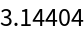#Function Repository Resource:

Compute the radical inverse of an integer to a given base

Contributed by: Jan Mangaldan

## Details

The radical inverse is also known as the van der Corput sequence.
Integer mathematical function, suitable for both symbolic and numerical manipulation.
The base-b radical inverse of n is defined as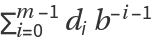, where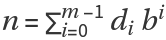is the base-b expansion of n, and m is IntegerLength[n,b].
The radical inverse is usually used for computing Halton and Hammersley low-discrepancy sequences for quasi-Monte Carlo methods.

## Examples

### Basic Examples (3)

The base-10 radical inverse of 42:

 In:=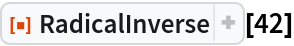Out=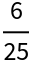The base-2 radical inverse of 42:

 In:=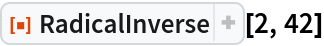Out=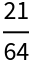In:=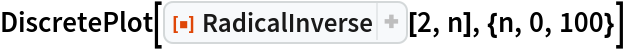Out=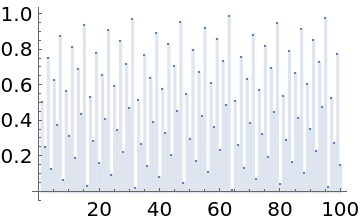### Scope (2)

Evaluate for large arguments:

 In:=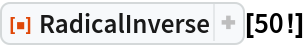Out=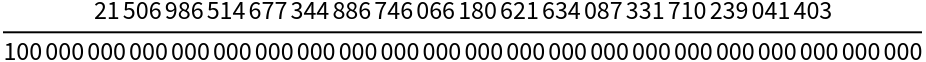In:=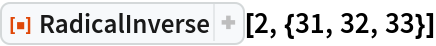Out=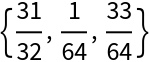### Applications (4)

Demonstrate the filling of the unit interval with the decimal radical inverse, also known as the van der Corput sequence:

 In:=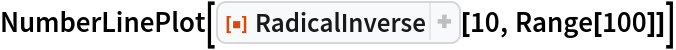Out=Generate a 2D Halton sequence with bases 2 and 3:

 In:=Out=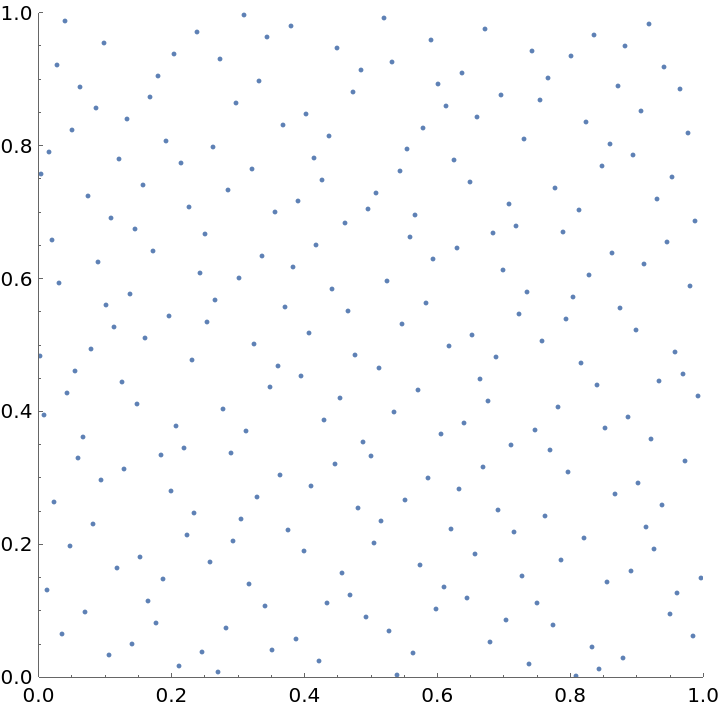Generate a 2D binary Hammersley sequence:

 In:=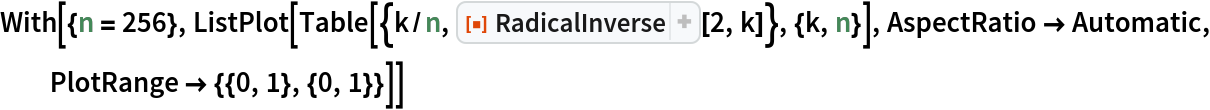Out=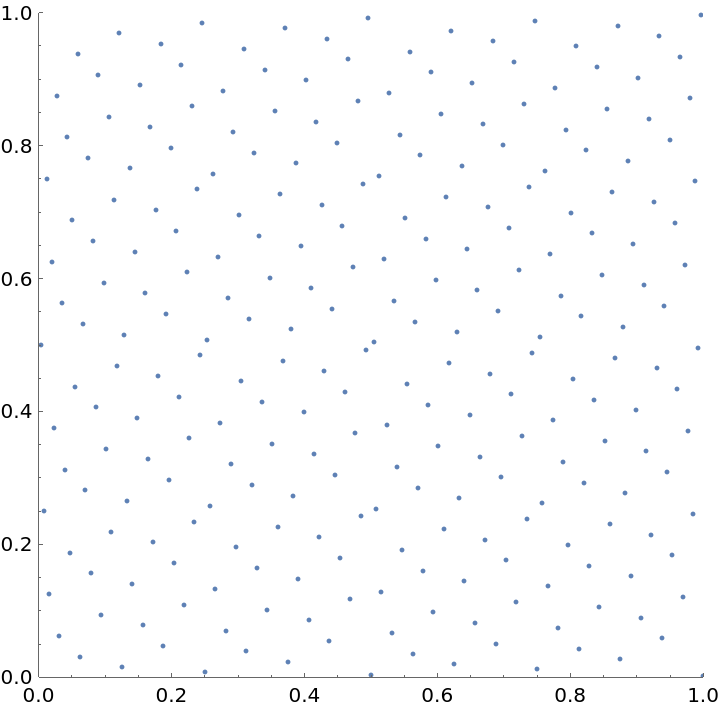Compare the Halton and Hammersley sequences for approximating π by quasi-Monte Carlo integration:

 In:=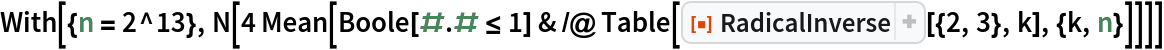Out=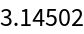In:=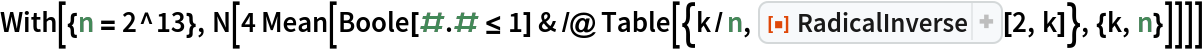Out=